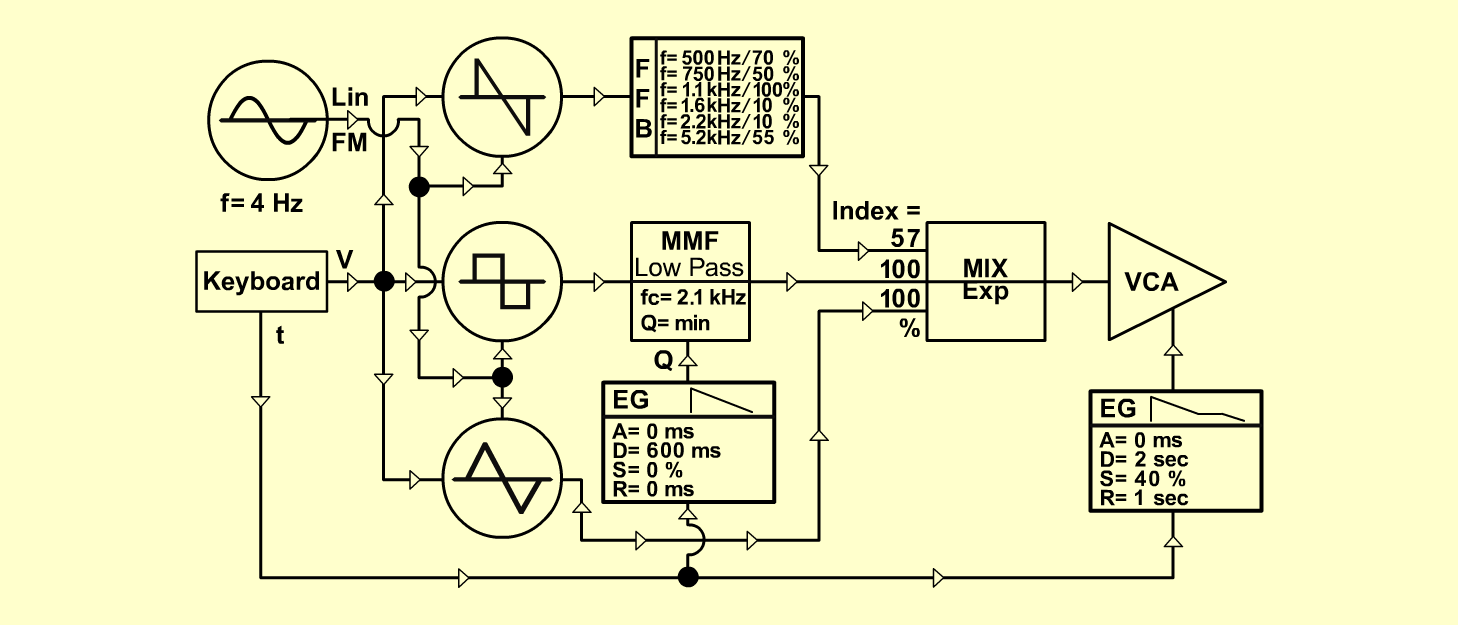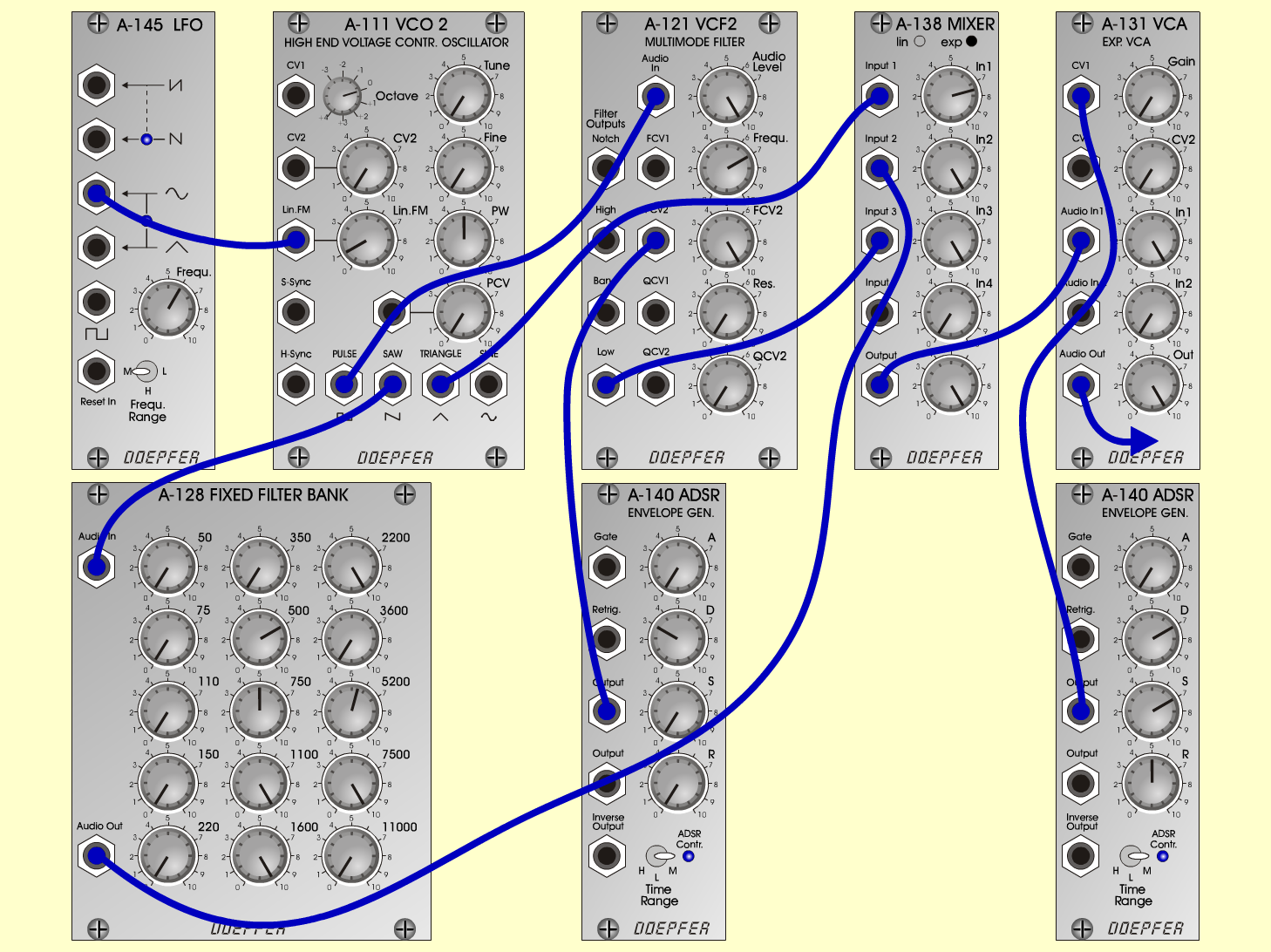HarpsichordKeyboardsD o e p f e r A - 100 Connections: Settings: A-145 (Sine)  <=>  A-111 (Lin FM)   A-111 (Square)  <=>  A-121 (Audio In)   A-111 (Saw)  <=>   A-128 (In)   A-111 (Triangle)  <=>  A-138 (Input 1)   A-128 (Out)  <=>  A-138 (Input 2)   A-121 (Low)  <=>  A-138 (Input 3)   A-140/1 (Output)  <=>  A-121 (FCV 2)   A-140/2 (Output)  <=>  A-131 (CV 1)   A-138 (Out)  <=>  A-131 (Audio In 1)   A-131 (Audio Out) <=> Amplifier A-145 (Frq = 6, Range = M)   A-111 (Lin FM = 1, PW = 5)   A-128 (50 = 0, 75 = 0, 110 = 0, 150 = 0, 220 = 0,   350 = 0, 700 = 7, 750 = 5, 1100 = 10, 1600 = 10,   2220 = 10, 3600 = 0, 5220 = 5.5, 7700 = 10,   11000=0)   A-121 (Audio Level = 10, Freq = 7, FCV 2 = 10,   Res = 0)   A-138 (In 1 = 7.5, In 2 = 10, In 3 = 10, Out = 10)   A-140/1 (A = 0, D = 3, S = 0, R = 0, Range = M)   A-140/2 (A = 0, D = 7, S = 7, R = 5, Range = M)    A-131 (Gain = 0, Audio In 1 = 10,  Audio Out = 10) Josef MuellerSound samples Bach: 3. Goldberg-Variation (BWV 988) Pachelbel: Canon in D     2./3. inst.: Strings / Moon-Walker Harpsichord